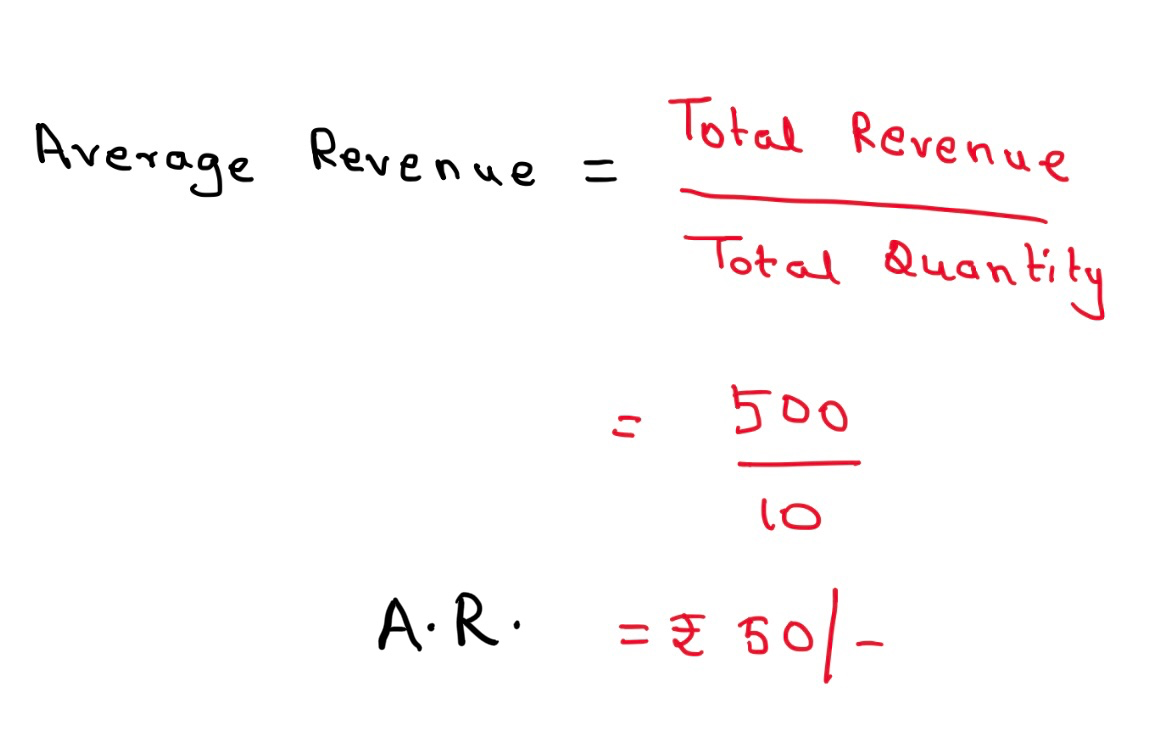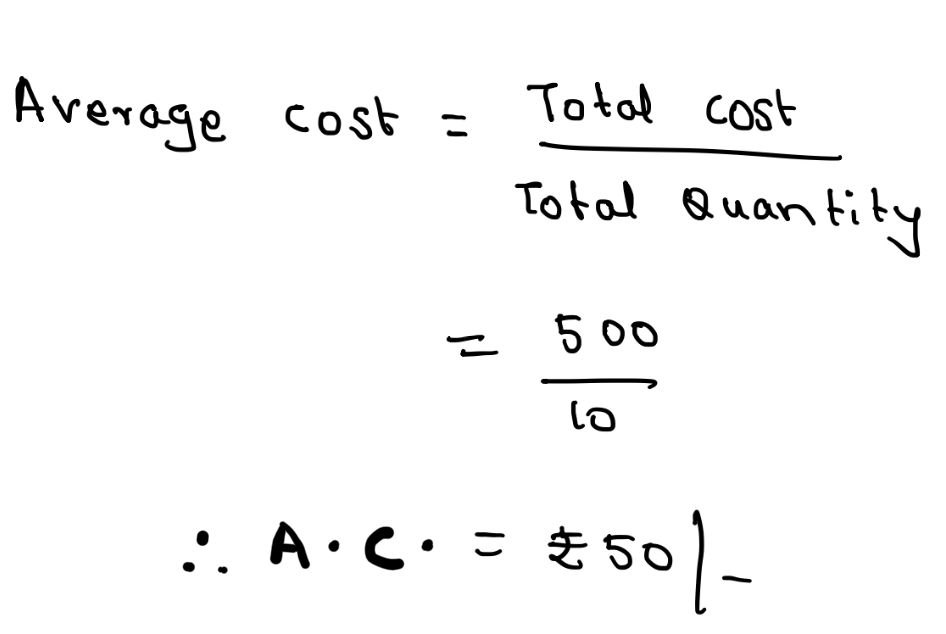### Average Revenue and Average cost

Average revenue refers to revenue received per unit from the sale of given quantity of goods. It is obtained by dividing total revenue by the number of units sold.
For example, if a producer sells 10 units of goods and receives total revenue of Rs. 500 then average revenue can be calculated as follows:Average Cost refers to Cost incurred per unit of producmtion and distribution. It is obtained by dividing total cost by the number of units produced.
For Example: If a producer produces 10 units of goods and incurred total cost of Rs. 500 then average cost can be calculated as follows.#### 1 comment:

1.